# KR100938087B1 - Method and apparatus for controlling reverse data rate in mobile communication system for packet data service - Google Patents

Method and apparatus for controlling reverse data rate in mobile communication system for packet data service Download PDF

## Info

Publication number
KR100938087B1
KR100938087B1 KR20030001727A KR20030001727A KR100938087B1 KR 100938087 B1 KR100938087 B1 KR 100938087B1 KR 20030001727 A KR20030001727 A KR 20030001727A KR 20030001727 A KR20030001727 A KR 20030001727A KR 100938087 B1 KR100938087 B1 KR 100938087B1
Authority
KR
South Korea
Prior art keywords
transition probability
transition
reverse
rate
set
Prior art date
Application number
KR20030001727A
Other languages
Korean (ko)
Other versions
KR20040064860A (en
Inventor
나석완
윤순영
허훈
Original Assignee
삼성전자주식회사
Priority date (The priority date is an assumption and is not a legal conclusion. Google has not performed a legal analysis and makes no representation as to the accuracy of the date listed.)
Filing date
Publication date
Application filed by 삼성전자주식회사 filed Critical 삼성전자주식회사
Priority to KR20030001727A priority Critical patent/KR100938087B1/en
Priority claimed from US10/754,602 external-priority patent/US20040213182A1/en
Publication of KR20040064860A publication Critical patent/KR20040064860A/en
Application granted granted Critical
Publication of KR100938087B1 publication Critical patent/KR100938087B1/en

• 230000001276 controlling effects Effects 0 abstract title 2
• 238000010295 mobile communication Methods 0 abstract title 2

## Images

••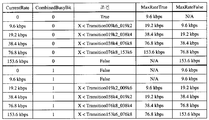•••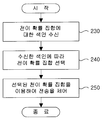•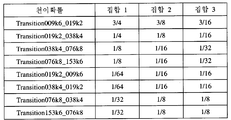•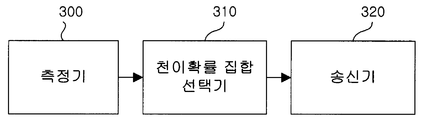•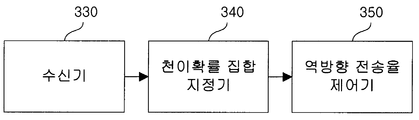•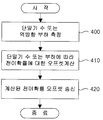••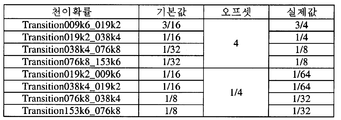•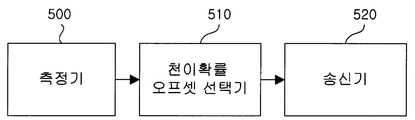•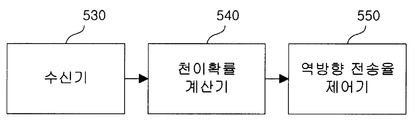• Y02B60/50

## Abstract

The present invention relates to a method and apparatus for determining a reverse data rate of a mobile communication system for a packet data service. The present invention relates to a maximum throughput while maintaining a reverse load below a reference value according to the number of terminals in a packet data service and a reverse load. An index indicating a set of transition probabilities that can be obtained or an offset to a reference set of transition probabilities are selected and transmitted to the terminal. Then, the terminal selects one transition probability corresponding to the current transmission rate from the set of transition probabilities indicated by the base station according to the reverse rate control information received from the base station, and predetermines the current transmission rate according to the selected transition probability. Loss is changed to one of a plurality of transmission rates. The present invention improves the throughput limited when the number of terminals is small or there is room in the reverse load, and enables efficient resource management by using the reverse load to the limit that can be accommodated in the reverse link.
Mobile Communication, 1x EV-DO, Reverse Rate Control, Transition Probability, Reverse Activity Bit (RAB), Throughput

## Description

Method and device for controlling reverse rate in mobile communication system supporting packet data service {METHOD AND APPARATUS FOR CONTROLLING REVERSE DATA RATE IN MOBILE COMMUNICATION SYSTEM FOR PACKET DATA SERVICE}

1 is a diagram illustrating a reverse traffic channel structure of an EV-DO according to the prior art.

2 is a condition table showing determination of reverse rate.

3 shows scheduling and transmit power parameters for throughput simulation of a reverse link.

4 is a flowchart illustrating an operation of a base station for controlling reverse rate using a plurality of transition probability sets according to the first embodiment of the present invention.

5 is a flowchart illustrating an operation of a terminal for controlling a reverse rate by using a plurality of transition probability sets according to the first embodiment of the present invention.

6 illustrates an example of transition probability sets.

7 illustrates a base station apparatus for controlling reverse rate using a plurality of transition probability sets according to the first embodiment of the present invention.

8 illustrates a terminal device for controlling a reverse rate using a plurality of transition probability sets according to a first embodiment of the present invention.

9 is a flowchart illustrating an operation of controlling a reverse rate by using one transition probability set and a transition probability offset according to the second embodiment of the present invention.

10 is a flowchart illustrating an operation of a terminal for controlling a reverse rate using one transition probability set and a transition probability offset according to the second embodiment of the present invention.

11 illustrates an example of a transition probability set and a transition probability offset.

12 is a diagram illustrating a base station apparatus for controlling a reverse rate by using one transition probability set and a transition probability offset according to a second embodiment of the present invention.

FIG. 13 is a diagram illustrating a terminal device for controlling a reverse rate using one transition probability set and a transition probability offset according to a second embodiment of the present invention. FIG.

The present invention relates to a mobile communication system, and more particularly, to a method and an apparatus for controlling a reverse rate in order to efficiently use a reverse link load in a packet data service.

As communication technologies have developed, more and more users want to use packet data services such as web, file transfer protocol (FTP), and video in a wireless environment. The traffic of such a packet data service is very asymmetrical. That is, the amount of traffic moving from the server providing the service to the user is very large compared to the amount of traffic moving from the user to the server. Considering the data service using mobile communication, since the server is connected to the user terminal through the base station, the amount of traffic on the forward link transmitted from the base station to the terminal is much larger than the amount of traffic on the reverse link transmitted from the terminal to the base station.

CDMA2000 1x Evolution-Data Only (EV-DO) or High Rate Packet Data Air interface (HRPDA), 1x Evolution-Data and Voice (EV-DV), 3GPP (3GPP2) of 3rd Generation Partnership Project 2 (3GPP2) Mobile communication standards such as HSDPA (High Speed Downlink Packet Access) of the 3rd Generation Partnership Project are designed in consideration of this asymmetric characteristic.

In particular, EV-DO uses high-order modulation schemes and coding rates such as 16-ary quadrature amplitude modulation (16-QAM) and 8-ary phase shift keying (8PSK) on the forward link. Adaptive Modulation and Coding Scheme (AMCS), Hybrid Automatic Repeat ReQuest (H-ARQ), and Multi-User Diversity Using Fat-Pipe Scheduling It is designed to achieve high throughput through the use of such techniques, etc. In addition, the reverse link employs scheduling and load control schemes considering the burst characteristics of packet data.

1 shows a structure of a reverse traffic channel used in a typical EV-DO system.

Referring to FIG. 1, the reverse traffic channel 100 includes a pilot channel 110, a medium access control (MAC) channel 120, an acknowledgment (ACK) channel 130, and a data channel 140. 120 is further divided into a reverse rate indicator (RRI) channel 122 and a data rate control (DRC) channel 124.

The pilot channel 110 is continuously transmitted for synchronization and channel estimation for the traffic channel. The RRI channel 122 sends an indicator indicating the rate of the traffic channel and the DRC channel 124 feeds back channel state information of the forward link for adaptive modulation and coding (AMSC) on the forward link. The ACK channel 130 transmits an ACK / NACK indicating whether the data packet received on the forward link is in error in order to support the complex-ARQ. Data channel 140 transmits a user's data packet.

The reverse traffic channel 100 is designed to support six transmission rates such as 9.6 kbps, 19.2 kbps, 38.4 kbps, 76.8 kbps, and 153.6 kbps. Scheduling is necessary to determine the reverse traffic channel rates of the terminals and control the load of the reverse link accordingly. In a mobile communication system before DV-DO, a base station performs scheduling based on feedback information received from terminals to determine transmission rates of the terminals and informs the terminals of the determined transmission rates.

Due to the large time delay, it is difficult to timely use traffic and channel information in consideration of the unexpected nature of packet data, and the load on the forward link is increased because information for reverse scheduling (ie, transmission rate) is transmitted through the forward link. There is a problem. Therefore, the EV-DO uses a method of increasing or decreasing the transmission rate in a probabilistic manner when the base station measures the reverse load on a frame basis and informs whether the transmission rate should be increased or decreased. To this end, the Reverse Activity Bit (RAB), Transition Probability, and Rate Limit parameters are used.

The RAB is 1 bit of information included in every forward frame and indicates to increase or decrease the reverse rate. The base station controls the reverse load by observing the reverse link load and setting the RAB appropriately. That is, when the load of the reverse link is lower than the preset reference value, the RAB is set to '0' to increase the transmission rate, and when the reverse link is higher than the reference value, the RAB is set to '1' to lower the transmission rate.

The transition probability includes a probability of transitioning to an upper transmission rate and a transition rate to a lower transmission rate for each transmission rate. The terminal generates a random number between 0 and 1 when determining the rate of the reverse traffic channel and converts the random number to a higher transmission rate according to RAB (hereinafter referred to as a higher transition probability) or to a lower transmission rate. Change or maintain the current rate in comparison with (hereinafter referred to as lower transition probability).

The upper transition probability is expressed as Transition009k6_019k2, Transition019k2_038k4, Transition038k4_076k8, and Transition076k8_153k6 depending on the current transmission rate. Typically, Transition019k2_038k4 is a transition probability that can be increased to 38.4kbps when the current transmission rate is 19.2kbps. If the RAB received from the base station is '0', the generated random number is compared with the higher transition probability corresponding to the current transmission rate. If the terminal is smaller than this, the terminal transitions to the next higher transmission rate and otherwise maintains the current transmission rate.

The lower transition probability is expressed as Transition019k2_009k6, Transition038k4_019k2, Transition076k8_038k4 and Transition153k6_076k8 according to the current transmission rate. If the RAB received from the base station is '1', the generated random number is compared with a lower transition probability corresponding to the current transmission rate. If the terminal is smaller than this, the terminal transitions to the next lower transmission rate and otherwise maintains the current transmission rate.

The RateLimit parameter is the maximum rate that a terminal can transmit on the reverse link, determined by the base station and periodically (for example every 768 slots) to all terminals in data service over a synchronous control channel. Per broadcast) or transmitted per terminal using a separate designated signal.

Referring to the scheduling procedure by the terminal for controlling the reverse rate using the above parameters as follows. Hereinafter, the current rate indicates the transmission rate of the frame before scheduling (zero at the first transmission), the maximum rate indicates the maximum rate that can be allocated after the scheduling, and the new rate is determined by the scheduling. Indicates the assigned rate.

1. The terminal ORs the RABs received from all base stations in communication by soft handoff to generate a combined busy bit (CBB).

2. The terminal generates a random number X having a uniform distribution between 0 and 1, and the condition given by the current transmission rate and the combined congestion bit is true for the generated random number with reference to the condition table shown in FIG. ), If true, the maximum rate is determined as the maximum rate, and if false, the maximum rate is determined as the maximum rate.

3. The new rate is set to the lesser of the determined maximum rate and the predetermined limit rate.

4. The terminal determines whether data transmission at the predetermined new transmission rate is possible according to the available transmission power, and if not, lowers the new transmission rate to the maximum transmission rate.

5. The terminal also determines whether the amount of user data to be transmitted is smaller than the amount that can be transmitted according to the predetermined new rate, and if it is small, lowers the new rate to the minimum rate that can transmit all of the amount of data that can be transmitted.

6. Transfer data at the new optimized data rate through 4.5 procedures.

For example, if the current rate is 19.2 kbps, the RABs received from all base stations in soft handoff are '0', the Transition019k2_038k4 value is 0.3, and the limited rate is 153.6 kbps, then the combined congestion bit (CBB) is' 0 ', so it corresponds to the fourth line of FIG. If the generated random number X is 0.2, the condition is true, so the maximum data rate is 38.4 kbps. If both of the above-mentioned transmission power and packet data amount conditions are satisfied, the new frame rate of the next frame is determined to be 38.4 kbps.

Meanwhile, the base station determines the RAB by measuring reverse load or rise over thermal (ROT). Herein, a method of using the reverse load will be described. When the base station uses a plurality of receive antennas, the reverse load Y j for the j th antenna is calculated as in Equation 1 below.

Where E_cp is the received energy of the pilot channel, I O is the total power spectral density, E C, DRC / E C, PILOT is the power ratio of the DRC channel to the pilot channel, and E C, DATA (R k ) / E C, PILOT is the power ratio of the data channel to the pilot channel when the k-th user's data rate is R k . The base station calculates the reverse load Z j for the j th antenna according to Equation 1 above, and sets RAB to '1' if the maximum value of the ROTs calculated for each antenna is greater than a predetermined reference value Y T. Otherwise set RAB to '0'.

Table 1 below shows the throughput simulation results of the reverse link using Equation 1. The scheduling parameters used here, namely the limited rate and transition probabilities and the transmit power parameters, E C, DRC / E C, PILOT and E C, DATA (R k ) / E C, PILOT are shown in FIG. 3. same. In addition, the ACK channel is neglected in the simulation because the ratio of the reverse load is very small.

Number of terminals Throughput (kbps) Load 4 248.02 0.48 8 267.73 0.61 12 250.06 0.63 16 217.82 0.88

As shown in FIG. 3, the higher the transmission rate of the EV-DO reverse link, the smaller the power ratio for the data channel of the overhead channel such as the pilot channel and the DRC channel, and the gain of the turbo code. Therefore, when the load is kept constant, a small number of terminals transmit at a high transmission rate, and a throughput is higher than a large number of terminals transmit at a low transmission rate. In other words, the smaller the number of terminals, the higher the throughput.

In the simulation results shown in Table 1, however, the throughput is lower than the number 8 when the number of terminals is four. On the other hand, in case of 4 terminals, the reverse load is much lower than the load reference value Y T (0.65625). This is because, even if there is a margin in the reverse load because the transition probability is fixed, the transmission rate of the terminal does not respond quickly when the reverse link state does not increase and the reverse link state improves.

In general, since the probability of transition to the higher rate is small and the probability of transition to the lower rate is large, it is difficult to raise the rate but easy to reduce it. The transition probability is fixed at a very conservative value in consideration of the worst case, that is, when the number of terminals is large. That is, the conventional system has a problem that the proper throughput cannot be obtained even when the number of terminals is small and there is room in the reverse load because the transition probability cannot be changed.

Therefore, the present invention devised to solve the problems of the prior art operating as described above provides a method and apparatus for controlling the reverse rate of the mobile communication system.

The present invention provides a method and apparatus for controlling the reverse rate by using the transition probability to increase the data throughput in the mobile communication system.

According to a first aspect of the present invention, there is provided a method of controlling a reverse rate by a base station in a mobile communication system that provides a packet data service to a terminal through a base station.

Measuring the number of terminals in a packet data service or a reverse load;

Selecting a transition probability set corresponding to the measured number of terminals or a reverse load from among a plurality of transmission probability sets predetermined as the terminal, wherein the transition probability sets are higher than a plurality of predetermined transmission rates; Each of the upper and lower transition probabilities representing the transition probability to the transmission rate and the lower transition probabilities representing the transition probability to the lower transmission rate, respectively.

And transmitting indication information on the selected transition probability set to the terminal in order for the terminal to control a reverse rate using the selected transition probability set.

According to a second aspect of the present invention, there is provided a method of controlling a reverse rate by a terminal in a mobile communication system that provides a packet data service to a terminal through a base station.

Receiving indication information on a set of transition probabilities from the base station to obtain a maximum throughput while maintaining a reverse load below a reference value;

Selecting one transition probability set corresponding to the received indication information from among a plurality of transmission probability sets equal to the base station, wherein the transition probability set is higher than a plurality of predetermined transmission rates; Each of the upper and lower transition probabilities representing the transition probability to the transmission rate and the lower transition probabilities representing the transition probability to the lower transmission rate, respectively.

A plurality of transmission rates for selecting one transition probability corresponding to a current transmission rate among the selected set of transition probabilities according to the reverse rate control information received from the base station, and for setting the current transmission rate according to the selected transition probability; It characterized in that it comprises the step of changing to one of the transmission rate.

According to a second aspect of the present invention according to the first aspect, a method of controlling a reverse rate by a base station in a mobile communication system providing a packet data service to a terminal through a base station,

Measuring the number of terminals in a packet data service or a reverse load;

Selecting a transition probability offset corresponding to the measured number of terminals or a reverse load with respect to the reference transition probability set predetermined as the terminal, wherein the transition probability set is an upper transmission rate among a plurality of predetermined transmission rates; It consists of upper and lower transition probabilities indicating the transition probability of the path and lower transition probabilities indicating the transition probability to the lower transmission rate,

And transmitting the offset of the selected transition probability set to the terminal so that the terminal controls the reverse rate by using the reference transition probability set and the selected transition probability offset.

According to a second aspect of the present invention according to a second aspect, a method of controlling a reverse rate by a terminal in a mobile communication system providing a packet data service to a terminal through a base station,

Receiving a transition probability offset of a transition probability set from the base station to obtain a maximum throughput while maintaining a reverse load below a reference value;

Updating a reference transition probability set predetermined with the base station with the received transition probability offset, wherein the transition probability set is an upper transition indicating a transition probability to a higher transmission rate among a plurality of predetermined transmission rates; Consists of probabilities and sub-transition probabilities representing the probability of transition to sub-rates,

Selecting a plurality of transition probabilities corresponding to a current transmission rate among the updated set of transition probabilities according to the reverse rate control information received from the base station, and determining the current transmission rate according to the selected transition probability. It characterized in that it comprises the step of changing to one of the transmission rate.

In the following description of the present invention, detailed descriptions of well-known functions or configurations will be omitted if it is determined that the detailed description of the present invention may unnecessarily obscure the subject matter of the present invention. Terms to be described later are terms defined in consideration of functions in the present invention, and may be changed according to intentions or customs of users or operators. Therefore, the definition should be made based on the contents throughout the specification.

The present invention described below is to control the transition rates to the upper and lower transmission rate in controlling the transmission rate of the reverse traffic channel in the mobile communication system supporting the packet data service.

The first embodiment of the present invention described below uses a different set of transition probabilities depending on the number of terminals and the load of the reverse link. In this case, the terminal has a plurality of transition probability sets, and when the base station designates an appropriate transition probability set according to the number of terminals or the load of the reverse link, the terminal controls the reverse rate using the designated transition probability set.

In addition, the second embodiment of the present invention uses an offset for one set of transition probabilities according to the number of terminals and the load amount of the reverse link. Here, when the terminal receives the transition probability set from the base station and the base station specifies an appropriate transition probability offset according to the number of terminals or the load of the reverse link, the terminal corrects the transition probabilities of the transition probability set according to the offset to obtain a reverse rate. Used to control.

Hereinafter, the first embodiment and the second embodiment of the present invention will be described in detail.

<< first embodiment >>

4 is a flowchart illustrating an operation of a base station for controlling a reverse rate by using a plurality of transition probability sets according to the first embodiment of the present invention. The operation shown here is performed by a base station that performs packet data service with one or more terminals in a cell. The base station performs simulation or calculation to maintain the maximum throughput while maintaining the reverse load below a reference value for each terminal number or reverse load. An index for a set of transition probabilities that can achieve is provided in advance. A transition probability set has upper and lower transition probabilities, and the upper transition probability indicates a transition probability to an upper transmission rate among a plurality of predetermined transmission rates, and the lower transition probability indicates a transition probability to a lower transmission rate.

Referring to FIG. 4, the base station continuously measures the number of terminals currently receiving packet data service or the load of the reverse link in step 200, and the measured terminals in step 210. Select the index of the corresponding set of transition probabilities depending on the number or load of the reverse link. In step 220, the base station transmits an index of the selected transition probability set to terminals in a cell through a common control channel or a dedicated control channel.

FIG. 5 is a flowchart illustrating an operation of a terminal for controlling a reverse rate in response to FIG. 4. In this case, the terminal has a plurality of sets of transition probabilities having upper and lower transition probabilities, respectively. The set of transition probabilities are previously specified by a mobile communication standard or received from a base station at call setup.

Referring to FIG. 5, the terminal receives an index of a transition probability set from a base station in step 230 and selects a corresponding transition probability set from a plurality of preset transition probability sets using the index in step 240. do. In step 250, the terminal controls the reverse rate using the selected transition probability.

4 and 5 are periodically repeated while the base station and the terminal proceed with the packet data service.

Table 2 below shows the results of the reverse throughput simulation according to the first embodiment. Here, the throughput calculation formula of Equation 1 mentioned above is used, and scheduling parameters, namely, limited transmission rate and transition probability and transmission power parameters, that is, E C, DRC / E C, PILOT and E C, DATA (R k The values shown in FIG. 3 are used as) / E C, PILOT and the terminal pre-stores three sets of transition probabilities as shown in FIG. 6.

Number of terminals Selected set Throughput (kbps) Reverse load Throughput growth rate compared to conventional method 4 Set 1 305.39 0.62 23.13% 8 Set 2 280.22 0.62 4.67% 12 Set 3 250.06 0.63 0.0% 16 Set 3 217.82 0.68 0.0%

Comparing Table 2 with Table 1, there is a significant improvement in throughput when the number of terminals in the cell is small, i.e. when there is room for reverse load. As described above, the first embodiment achieves higher throughput than when the number of terminals is large by fully utilizing the advantage of the high data rate possible when the number of terminals is small. Here, the reverse load is maintained at the same level as when the number of terminals is large, and a value close to 0.65625 used as a reference value in FIG. 3 is obtained. As described above, the first embodiment can achieve a throughput that cannot be reached due to the conservative limitation of the probability of transition when the number of terminals is small, and increase the efficiency of the throughput by using the load to an acceptable limit on the reverse link. have.

7 and 8 illustrate a base station and a terminal device respectively controlling reverse rate using a plurality of transition probability sets according to the first embodiment of the present invention.

Referring to FIG. 7, the measurer 300 continuously measures the number or reverse loads of terminals in a packet data service by the base station, and the transition probability set selector 310 corresponds to the measured number of terminals or reverse loads. Select the index for the most appropriate set of transition probabilities. The transmitter 320 transmits the selected index to the terminals in the cell in a message or signal having a predetermined predetermined format.

Referring to FIG. 8, the receiver 330 receives an index for a transition probability set from a base station, and the transition probability set designator 340 designates a transition probability set for reverse rate control according to the index. The reverse rate controller 350 then determines the rate of the reverse data channel using the transition probabilities of the specified set of transition probabilities.

<< 2nd Example >>

9 is a flowchart illustrating an operation of a base station for controlling a reverse rate by using a reference transition probability set and a transition probability offset according to the second embodiment of the present invention. The operation shown here is performed by a base station that performs packet data service with one or more terminals in a cell. The base station performs simulation or calculation to maintain the maximum throughput while maintaining the reverse load below a reference value for each terminal number or reverse load. It is provided with an offset of the transition probabilities that can achieve. The offset is for respectively or simultaneously adjusting the upper and lower transition probabilities of the reference transition probability set that the terminal already has.

Referring to FIG. 9, in step 400, the base station continuously measures the number of terminals currently receiving packet data service in its cell or the load of the reverse link, and the measured terminal in step 410. The corresponding transition probability offset is selected depending on the number or load of the reverse link. In step 420, the base station transmits the selected transition probability offset to terminals in the cell through a common control channel or a dedicated control channel.

FIG. 10 is a flowchart illustrating an operation of a terminal for controlling a reverse rate in response to FIG. 9. Here, the terminal has one reference transition probability set having upper and lower transition probabilities. The reference transition probability set is previously specified by a mobile communication standard or received from a base station at call setup.

Referring to FIG. 10, the terminal receives a transition probability offset from the base station in step 430, and updates the transition probabilities of the reference transition probability set previously used in step 440. In step 450, the terminal controls the reverse rate using the transition probability.

9 and 10 are periodically repeated while the base station and the terminal proceed with the packet data service.

An example of a reference transition probability set and an offset used in the second embodiment is shown in FIG. 11. In FIG. 11, the offset for the upper transition probability is 4, the offset for the lower transition probability is 1/4, and the transition columns are updated in the last column of FIG. 11 by the offset values. As shown, updating upper transition probabilities 3/16, 1 / 16,1 / 32,1 / 32 to offset 4 results in 3 / 4,1 / 4,1 / 8,1 / 8, and lower transition probability Updating these 1 / 16,1 / 16,1 / 8,1 / 8 to offset 1/4 results in 1 / 64,1 / 64,1 / 32,1 / 32.

Table 3 below shows other examples of offsets that may be selected by the base station according to the number of terminals.

Number of terminals Offset of upper transition probability Offset of lower transition probability 4 4 1/4 8 2 One 12 One One 16 One One

When the offset according to the number of terminals is allocated as shown in Table 1, it becomes the same as using different sets of transition probabilities according to the number of terminals.

12 and 13 illustrate a base station and a terminal apparatus for controlling a reverse rate by using one reference transition probability set and a transition probability offset according to the second embodiment of the present invention.

Referring to FIG. 12, the measurer 500 continuously measures the number or reverse loads of terminals in a packet data service service by the base station, and the transition probability offset selector 510 corresponds to the measured number of terminals or reverse loads. To select the most appropriate transition probability offset. The transmitter 520 transmits the selected transition probability offset to a terminal in a cell on a predetermined message or signal of a predetermined format.

Referring to FIG. 13, the receiver 530 receives a transition probability offset from a base station, and the transition probability calculator 540 updates the transition probabilities of the reference transition probability set that it has in advance by using the received transition probability offset. Calculate the probability of transition to be used. Reverse rate controller 550 uses the calculated transition probabilities to determine the rate of the reverse data channel.

Meanwhile, in the detailed description of the present invention, specific embodiments have been described, but various modifications are possible without departing from the scope of the present invention. Therefore, the scope of the present invention should not be limited to the described embodiments, but should be defined not only by the scope of the following claims, but also by those equivalent to the scope of the claims.

In the present invention operating as described in detail above, the effects obtained by the representative of the disclosed invention are briefly described as follows.

According to the present invention, the transition probability used to determine the transmission rate of the reverse data channel of the mobile communication system for packet data is appropriately changed according to the number of terminals in the cell or the reverse load, so that the number of terminals is small or load due to the transition probability fixed to a conservative value. Efficient resource management can be achieved by improving the reverse throughput, which is limited when there is a short time, and using the reverse load to the limit that can be accommodated in the reverse link.

## Claims (30)

1. A method of controlling a reverse rate by a base station in a mobile communication system providing a packet data service to a terminal through a base station,
Measuring the number of terminals in a packet data service or a reverse load;
Selecting a transition probability set corresponding to the measured number of terminals or a reverse load from among a plurality of transmission probability sets predetermined as the terminal, wherein the transition probability sets are higher than a plurality of predetermined transmission rates; Each of the upper and lower transition probabilities representing the transition probability to the transmission rate and the lower transition probabilities representing the transition probability to the lower transmission rate, respectively.
And transmitting indication information on the selected transition probability set to the terminal in order for the terminal to control the reverse rate using the selected transition probability set.
2. The method of claim 1, wherein the selecting of the transition probability set comprises:
And selecting a set of transition probabilities that can obtain a maximum reverse throughput while maintaining the reverse load below a reference value.
3. The method of claim 1, wherein the higher transition probabilities have a smaller value as the number of terminals or reverse loads corresponding to the corresponding transition probability set is larger, and the lower transition probabilities are larger as the received signal-to-noise ratio corresponding to the corresponding transition probability set is larger. The reverse rate control method characterized in that it has a value.
4. The method of claim 1, wherein the indication information is transmitted through a common control channel or a dedicated control channel.
5. A method of controlling a reverse rate by a terminal in a mobile communication system providing a packet data service to a terminal through a base station,
Receiving indication information on a set of transition probabilities from the base station to obtain a maximum throughput while maintaining a reverse load below a reference value;
Selecting one transition probability set corresponding to the received indication information from among a plurality of transmission probability sets equal to the base station, wherein the transition probability set is higher than a plurality of predetermined transmission rates; Each of the upper and lower transition probabilities representing the transition probability to the transmission rate and the lower transition probabilities representing the transition probability to the lower transmission rate, respectively.
A plurality of transmission rates for selecting one transition probability corresponding to a current transmission rate among the selected set of transition probabilities according to the reverse rate control information received from the base station, and for setting the current transmission rate according to the selected transition probability; The reverse rate control method comprising the step of changing to one of the rate.
6. The method of claim 5, wherein the changing of the current transmission rate comprises:
If the reverse rate control information indicates congestion of the reverse link, one lower transition probability corresponding to the current rate is selected from the selected set of transition probabilities, so that random numbers generated with a uniform distribution between 0 and 1 are selected. Less than the transition probability, the current rate is reduced to a lower rate indicated by the selected lower transition rate,
If the reverse rate control information does not indicate congestion of the reverse link, one of the selected sets of transition probabilities corresponding to the current rate is selected, and the random number generated with a uniform distribution between 0 and 1 is selected. If less than an upper transition probability, increasing the current transmission rate to an upper transmission rate indicated by the selected upper transition probability.
7. 6. The method of claim 5, wherein the indication information is received through a common control channel or a dedicated control channel.
8. A base station apparatus for controlling a reverse rate in a mobile communication system that provides a packet data service to a terminal through a base station,
A measuring device for measuring the number of terminals in a packet data service or a reverse load;
A selector for selecting a transition probability set corresponding to the measured number of terminals or a reverse load from among a plurality of transmission probability sets predetermined as the terminal, wherein the transition probability sets are higher among a plurality of predetermined transmission rates Each of the upper and lower transition probabilities representing the transition probability to the transmission rate and the lower transition probabilities representing the transition probability to the lower transmission rate, respectively.
And a transmitter for transmitting the indication information on the selected transition probability set to the terminal in order for the terminal to control a reverse rate using the selected transition probability set.
9. The method of claim 8, wherein the selector,
And selecting a set of transition probabilities that can obtain a maximum throughput while maintaining the reverse load below a reference value.
10. 9. The method of claim 8, wherein the higher transition probabilities have a smaller value as the number of terminals or reverse loads corresponding to the set of transition probabilities increases, and the lower transition probabilities have a larger value as a received signal-to-noise ratio corresponding to the corresponding set of transition probabilities. Base station apparatus characterized in that it has a value.
11. The base station apparatus according to claim 8, wherein the indication information is transmitted through a common control channel or a dedicated control channel.
12. A terminal apparatus for controlling a reverse rate in a mobile communication system that provides a packet data service to a terminal through a base station,
A receiver for receiving indication information on a set of transition probabilities that can obtain a maximum throughput while maintaining a reverse load from the base station below a reference value;
A selector for selecting one transition probability set corresponding to the received indication information from among a plurality of transmission probability sets equal to the base station, wherein the transition probability sets are higher than a plurality of predetermined transmission rates; Each of the upper and lower transition probabilities representing the transition probability to the transmission rate and the lower transition probabilities representing the transition probability to the lower transmission rate, respectively.
A plurality of transmission rates for selecting one transition probability corresponding to a current transmission rate among the selected set of transition probabilities according to the reverse rate control information received from the base station, and for setting the current transmission rate according to the selected transition probability; And a reverse rate controller for changing one of the rate rates.
13. The method of claim 12, wherein the reverse rate controller,
If the reverse rate control information indicates congestion of the reverse link, one lower transition probability corresponding to the current rate is selected from the selected set of transition probabilities, so that random numbers generated with a uniform distribution between 0 and 1 are selected. Less than the transition probability, the current rate is reduced to a lower rate indicated by the selected lower transition rate,
If the reverse rate control information does not indicate congestion of the reverse link, one of the selected sets of transition probabilities corresponding to the current rate is selected, and the random number generated with a uniform distribution between 0 and 1 is selected. Less than an upper transition probability, increases the current transmission rate to an upper transmission rate indicated by the selected upper transition probability.
14. The terminal device of claim 12, wherein the indication information is received through a common control channel or a dedicated control channel.
15. A method of controlling a reverse rate by a base station in a mobile communication system providing a packet data service to a terminal through a base station,
Measuring the number of terminals in a packet data service or a reverse load;
Selecting a transition probability offset corresponding to the measured number of terminals or a reverse load with respect to the reference transition probability set predetermined as the terminal, wherein the transition probability set is an upper transmission rate among a plurality of predetermined transmission rates; It consists of upper and lower transition probabilities indicating the transition probability of the path and lower transition probabilities indicating the transition probability to the lower transmission rate,
And transmitting the offset of the selected transition probability set to the terminal so that the terminal controls the reverse rate using the reference transition probability set and the selected transition probability offset.
16. The method of claim 15, wherein the selecting of the offset of the transition probability set comprises:
And selecting a transition probability offset of the set of transition probabilities for obtaining the maximum throughput while maintaining the reverse load at or below the reference value with respect to the reference transition probability set.
17. The method of claim 15, wherein the offset of the set of transition probabilities,
And an offset for the upper transition probabilities and an offset for the lower transition probabilities.
18. The method of claim 17,
The offset for the higher transition probabilities has a value for further reducing the upper transition probabilities as the number of corresponding terminals or the reverse load is larger,
And the offset for the lower transition probabilities has a value for further increasing the lower transition probabilities as the number of corresponding terminals or the reverse load increases.
19. 16. The method of claim 15, wherein the offset is transmitted through a common control channel or a dedicated control channel.
20. A method of controlling a reverse rate by a terminal in a mobile communication system providing a packet data service to a terminal through a base station,
Receiving a transition probability offset of a transition probability set from the base station to obtain a maximum throughput while maintaining a reverse load below a reference value;
Updating a reference transition probability set predetermined with the base station with the received transition probability offset, wherein the transition probability set is an upper transition indicating a transition probability to a higher transmission rate among a plurality of predetermined transmission rates; Consists of probabilities and sub-transition probabilities representing the probability of transition to sub-rates,
Selecting a plurality of transition probabilities corresponding to a current transmission rate among the updated set of transition probabilities according to the reverse rate control information received from the base station, and determining the current transmission rate according to the selected transition probability. The reverse rate control method comprising the step of changing to one of the rate.
21. The method of claim 20, wherein changing the current transmission rate comprises:
If the reverse rate control information indicates congestion of the reverse link, one lower transition probability corresponding to the current rate is selected from the updated set of transition probabilities, and the random number generated with a uniform distribution between 0 and 1 is selected. If the lower transition probability is less than the current transmission rate is reduced to the lower transmission rate indicated by the selected lower transition probability,
If the reverse rate control information does not indicate congestion of the reverse link, one upper transition probability corresponding to the current rate is selected from the updated set of transition probabilities, and the random number generated with a uniform distribution between 0 and 1 is selected. If less than the selected higher transition probability, increasing the current transmission rate to an upper transmission rate indicated by the selected upper transition probability.
22. 21. The method of claim 20, wherein the offset is received through a common control channel or a dedicated control channel.
23. A base station apparatus for controlling a reverse rate in a mobile communication system that provides a packet data service to a terminal through a base station,
A measuring device for measuring the number of terminals in a packet data service or a reverse load;
A selector for selecting a transition probability offset of a transition probability set corresponding to the measured number of terminals or a reverse load with respect to a predetermined reference transition probability set equal to the terminal, wherein the transition probability set is a plurality of predetermined transmission rates Among them, it is composed of upper transition probabilities indicating transition probability to higher transmission rate and lower transition probabilities indicating transition probability to lower transmission rate,
And a transmitter for transmitting an offset of the selected transition probability set to the terminal in order for the terminal to control a reverse rate using the reference transition probability set and the selected transition probability offset.
24. The method of claim 23, wherein the selector,
And a transition probability offset of a transition probability set for obtaining a maximum throughput while maintaining the reverse load below a reference value with respect to the reference transition probability set.
25. The method of claim 23, wherein the offset of the set of transition probabilities is
And an offset for the upper transition probabilities and an offset for the lower transition probabilities.
26. The method of claim 25,
The offset for the higher transition probabilities has a value for further reducing the upper transition probabilities as the number of corresponding terminals or the reverse load is larger,
And the offset for the lower transition probabilities has a value for further increasing the lower transition probabilities as the number of corresponding terminals or the reverse load increases.
27. The base station apparatus according to claim 23, wherein the offset is transmitted through a common control channel or a dedicated control channel.
28. A terminal apparatus for controlling a reverse rate in a mobile communication system that provides a packet data service to a terminal through a base station,
A receiver for receiving a transition probability offset of a set of transition probabilities from the base station to obtain a maximum throughput while maintaining a reverse load below a reference value;
A calculator for updating a reference transition probability set predetermined with the received transition probability offset in the same manner as the base station, wherein the transition probability set is an upper transition indicating a transition probability to a higher transmission rate among a plurality of predetermined transmission rates; Consists of probabilities and sub-transition probabilities representing the probability of transition to sub-rates,
Selecting a plurality of transition probabilities corresponding to a current transmission rate among the updated set of transition probabilities according to the reverse rate control information received from the base station, and determining the current transmission rate according to the selected transition probability. Terminal device comprising a reverse rate controller for changing to one of the rate.
29. 29. The apparatus of claim 28, wherein the reverse rate controller is:
If the reverse rate control information indicates congestion of the reverse link, one lower transition probability corresponding to the current rate is selected from the updated set of transition probabilities, and the random number generated with a uniform distribution between 0 and 1 is selected. If the lower transition probability is less than the current transmission rate is reduced to the lower transmission rate indicated by the selected lower transition probability,
If the reverse rate control information does not indicate congestion of the reverse link, one upper transition probability corresponding to the current rate is selected from the updated set of transition probabilities, and the random number generated with a uniform distribution between 0 and 1 is selected. If less than the selected higher transition probability, increasing the current transmission rate to an upper transmission rate indicated by the selected upper transition probability.
30. 29. The terminal apparatus of claim 28, wherein the offset is received through a common control channel or a dedicated control channel.
KR20030001727A 2003-01-10 2003-01-10 Method and apparatus for controlling reverse data rate in mobile communication system for packet data service KR100938087B1 (en)

## Priority Applications (1)

Application Number Priority Date Filing Date Title
KR20030001727A KR100938087B1 (en) 2003-01-10 2003-01-10 Method and apparatus for controlling reverse data rate in mobile communication system for packet data service

## Applications Claiming Priority (2)

Application Number Priority Date Filing Date Title
KR20030001727A KR100938087B1 (en) 2003-01-10 2003-01-10 Method and apparatus for controlling reverse data rate in mobile communication system for packet data service
US10/754,602 US20040213182A1 (en) 2003-01-10 2004-01-12 Apparatus and method for controlling a reverse rate in a mobile communication system supporting packet data service

## Publications (2)

Publication Number Publication Date
KR20040064860A KR20040064860A (en) 2004-07-21
KR100938087B1 true KR100938087B1 (en) 2010-01-21

# Family

## Family Applications (1)

Application Number Title Priority Date Filing Date
KR20030001727A KR100938087B1 (en) 2003-01-10 2003-01-10 Method and apparatus for controlling reverse data rate in mobile communication system for packet data service

## Country Status (1)

KR (1) KR100938087B1 (en)

## Families Citing this family (1)

* Cited by examiner, † Cited by third party
Publication number Priority date Publication date Assignee Title
KR100747691B1 (en) * 2006-04-21 2007-08-02 에스케이 텔레콤주식회사 Method and mobile terminal for providing reverse link data transmission for ev-do network by using payload

## Citations (4)

* Cited by examiner, † Cited by third party
Publication number Priority date Publication date Assignee Title
KR20020001659A (en) * 2000-06-28 2002-01-09 윤종용 Reverse data transmission method and apparatus in mobile communication system
KR20020032732A (en) * 2000-10-27 2002-05-04 구자홍 Transmission speed control method and device of reverse traffic channel of wireless data transmisson system
KR20020085140A (en) * 2001-05-04 2002-11-16 엘지전자 주식회사 Method for controling the data rate of reverse link, Terminal for the same

## Patent Citations (4)

* Cited by examiner, † Cited by third party
Publication number Priority date Publication date Assignee Title
KR20020001659A (en) * 2000-06-28 2002-01-09 윤종용 Reverse data transmission method and apparatus in mobile communication system
KR20020032732A (en) * 2000-10-27 2002-05-04 구자홍 Transmission speed control method and device of reverse traffic channel of wireless data transmisson system
KR20020085140A (en) * 2001-05-04 2002-11-16 엘지전자 주식회사 Method for controling the data rate of reverse link, Terminal for the same

## Also Published As

Publication number Publication date
KR20040064860A (en) 2004-07-21

## Similar Documents

Publication Publication Date Title
ES2672374T3 (en) Selection of transport format combination in a wireless transmission / reception unit
CN1708923B (en) Method and apparatus for providing a distributed architecture digital wireless communication system
DE60308457T2 (en) Adaptive modulation and coding
KR100828800B1 (en) Hybrid tdm/ofdm/cdm reverse link transmission
KR101273412B1 (en) Base station, mobile station, and power control method
CN1178548C (en) Closed loop resource allocation in high speed wireless communications network
US6487394B1 (en) Radio communication device and method of controlling transmission rate
JP5255473B2 (en) Transmission in a communication system
US7715347B2 (en) Method of enabling a combined data rate control lock channel in a wireless communication system
JP4435983B2 (en) Method and apparatus for distributed optimal reverse link scheduling of resources such as rate and power in a wireless communication system
EP1811804B1 (en) Controlling data transmission rate on the reverse link for each mobile station in a dedicated manner
KR100747365B1 (en) Transmission rate changes in communications networks
CN1225846C (en) Power control algorithm for packet data based on queuing/channel utilization
EP1207644B1 (en) Method of link adaptation of blind type using acknowledgements in ARQ system
JP4720838B2 (en) Communication apparatus and method, information processing apparatus and method
CA2408619C (en) Method and apparatus for fast closed-loop rate adaptation in a high rate packet data transmission
DE602004007853T2 (en) Control of transmission power from forward access channel (FACH), and corresponding network for mobile telecommunication
JP4879956B2 (en) Apparatus and method for transmitting uplink packet data in a mobile communication system
US7239847B2 (en) Method and apparatus for adaptive server selection in a data communication system
KR100651450B1 (en) Method for controlling of reverse link in a mobile communication system
CN1302627C (en) Method and apparatus for power control in wireless communication system
RU2327289C2 (en) Method and device for disconnected adaptive power control
EP1505756B1 (en) Adaptive modulation and coding

## Legal Events

Date Code Title Description
A201 Request for examination
E902 Notification of reason for refusal
E701 Decision to grant or registration of patent right
GRNT Written decision to grant
FPAY Annual fee payment

Payment date: 20121228

Year of fee payment: 4

FPAY Annual fee payment

Payment date: 20131230

Year of fee payment: 5

FPAY Annual fee payment

Payment date: 20141223

Year of fee payment: 6

FPAY Annual fee payment

Payment date: 20151229

Year of fee payment: 7

FPAY Annual fee payment

Payment date: 20161228

Year of fee payment: 8

LAPS Lapse due to unpaid annual fee